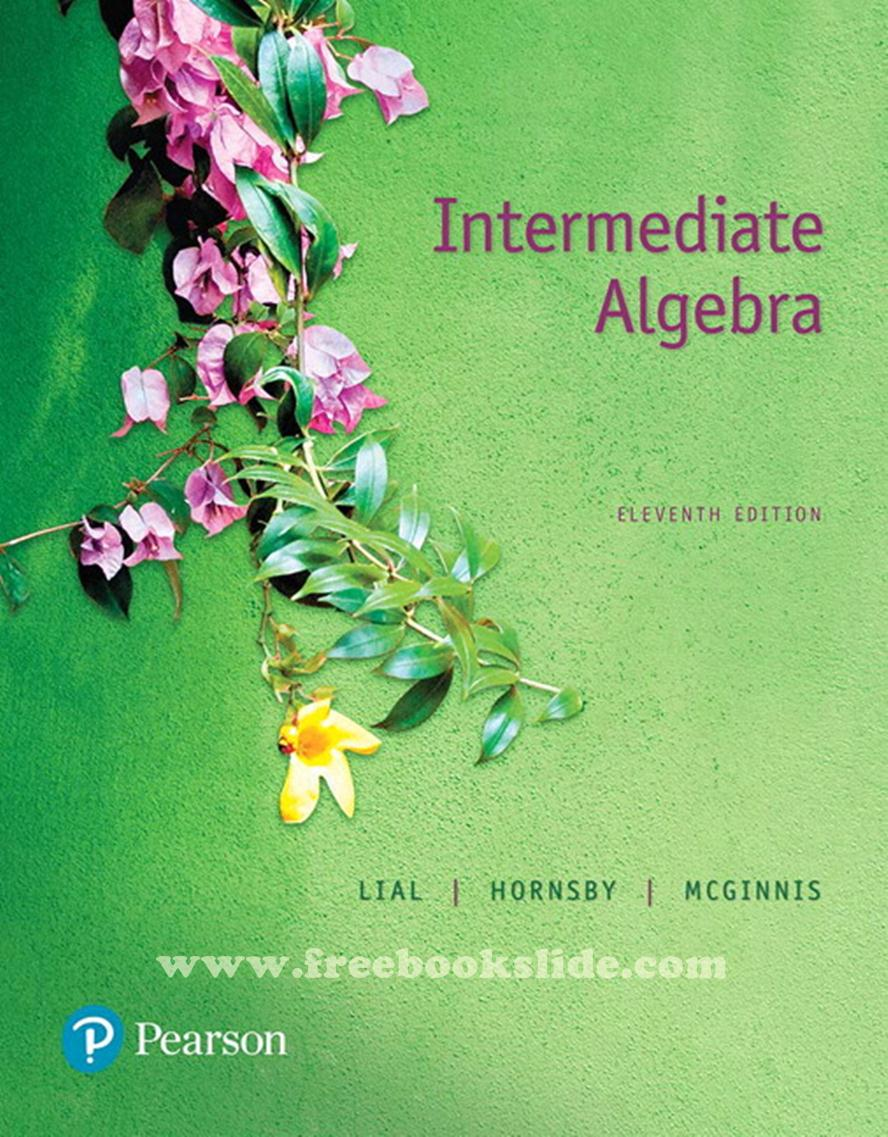### Ebook Intermediate Algebra 11e by Lial, Hornsby, McGinnisIntermediate Algebra
11th Edition
Margaret L. Lial - John Hornsby - Terry McGinnis
Pea*son Ed*cation

Table of Content:
R. Review of the Real Number System
R.1 Basic Concepts
R.2 Operations on Real Numbers
R.3 Exponents, Roots, and Order of Operations
R.4 Properties of Real Numbers
Study Skills Using Your Math Text
1. Linear Equations and Applications
1.1 Linear Equations in One Variable
1.2 Formulas and Percent
1.3 Applications of Linear Equations
Study Skills Taking Lecture Notes
1.4 Further Applications of Linear Equations
Summary Exercises Applying Problem-Solving Techniques
Study Skills Reviewing a Chapter
2. Linear Inequalities and Absolute Value
2.1 Linear Inequalities in One Variable
Study Skills Using Study Cards
2.2 Set Operations and Compound Inequalities
Study Skills Using Study Cards Revisited
2.3 Absolute Value Equations and Inequalities
Summary Exercises Solving Linar and Absolute Value Equations and Inequalities
Study Skills Taking Math Tests
3. Graphs, Linear Equations and Functions
3.1 The Rectangular Coordinate System
Study Skills Analyzing Your Test Results
3.2 Slope of a Line
3.3 Linear Equations in Two Variables
Summary Exercises Finding Slopes and Equations of Lines
3.4 Linear Inequalities in Two Variables
3.5 Introduction to Relations and Functions
3.6 Function Notation and Linear Functions
4. Systems of Linear Equations
4.1 Systems of Linear Equations in Two Variables
Study Skills Preparing for Your Math Final Exam
4.2 Systems of Linear Equations in Three Variables
4.3 Applications of Systems of Linear Equations
5. Exponents, Polynomials, and Polynomial Functions
5.1 Integer Exponents
5.2 Scientific Notation
5.4 Polynomial Functions and Graphs
5.5 Multiplying Polynomials
5.6 Dividing Polynomials
6. Factoring
6.1 Greatest Common Factors and Factoring by Grouping
6.2 Factoring Trinomials
6.3 Special Factoring
6.4 A General Approach to Factoring
6.5 Solving Equations Using the Zero-Factor Property
7. Rational Expressions and Functions
7.1 Rational Expressions and Functions; Multiplying and Dividing
7.2 Adding and Subtracting Rational Expressions
7.3 Complex Fractions
7.4 Equations with Rational Expressions and Graphs
Summary Exercises Simplifying Rational Expressions vs. Solving Rational Equations
7.5 Applications of Rational Expressions
7.6 Variation
8. Roots, Radicals, and Root Functions
8.2 Rational Exponents
8.5 Multiplying and Dividing Radical Expressions
Summary Exercises Performing Operations with Radicals and Rational Exponents
8.7 Complex Numbers
9. Quadratic Equations, Inequalities, and Functions
9.1 The Square Root Property and Completing the Square
Summary Exercises Applying Methods for Solving Quadratic Equations
9.4 Formulas and Further Applications
9.6 More about Parabolas and Their Applications
9.7 Polynomial and Rational Inequalities
10. Inverse, Exponential, and Logarithmic Functions
10.1 Composition of Functions
10.2 Inverse Functions
10.3 Exponential Functions
10.4 Logarithmic Functions
10.5 Properties of Logarithms
10.6 Common and Natural Logarithms
10.7 Exponential and Logarithmic Equations and Their Applications
11. Nonlinear Functions, Conic Sections, and Nonlinear Systems